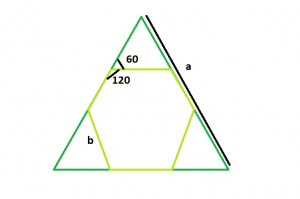# Largest hexagon that can be inscribed within an equilateral triangle

Given an equilateral triangle of side length a, the task is to find the largest hexagon that can be inscribed within it.

Examples:

Input: a = 6
Output: 2

Input: a = 9
Output: 3

## Recommended: Please try your approach on {IDE} first, before moving on to the solution.Approach: From the figure, it is clear that the three small triangles are also equilateral. So they will have side length b = a / 3 where b is also the length of the hexagon and a is the length of the given equilateral triangle.

Below is the implementation of the above approach:

## C++

 `// C++ program to find the side of the ` `// largest hexagon which can be inscribed ` `// within an equilateral triangle ` `#include ` `using` `namespace` `std; ` ` `  `// Function to find the side ` `// of the hexagon ` `float` `hexagonside(``float` `a) ` `{ ` ` `  `    ``// Side cannot be negative ` `    ``if` `(a < 0) ` `        ``return` `-1; ` ` `  `    ``// Side of the hexagon ` `    ``float` `x = a / 3; ` `    ``return` `x; ` `} ` ` `  `// Driver code ` `int` `main() ` `{ ` `    ``float` `a = 6; ` `    ``cout << hexagonside(a) << endl; ` `    ``return` `0; ` `} `

## Java

 `// Java program to find the side of the ` `// largest hexagon which can be inscribed ` `// within an equilateral triangle ` `class` `CLG ` `{ ` `// Function to find the side ` `// of the hexagon ` ` ``static` `float` `hexagonside(``float` `a) ` `{ ` ` `  `    ``// Side cannot be negative ` `    ``if` `(a < ``0``) ` `        ``return` `-``1``; ` ` `  `    ``// Side of the hexagon ` `    ``float` `x = a / ``3``; ` `    ``return` `x; ` `} ` ` `  `// Driver code ` `public` `static` `void` `main(String[] args) ` `{ ` `    ``float` `a = ``6``; ` `    ``System.out.println(hexagonside(a)); ` `     `  `} ` `} `

## Python3

 `# Python3 program to find the side of the ` `# largest hexagon which can be inscribed  ` `# within an eqilateral triangle ` ` `  `# function to find the side of the hexagon ` `def` `hexagonside(a): ` `     `  `    ``# Side cannot be negative ` `    ``if` `a < ``0``: ` `        ``return` `-``1` `         `  `    ``# Side of the hexagon ` `    ``x ``=` `a ``/``/` `3` `    ``return` `x ` ` `  `# Driver code ` `a ``=` `6` `print``(hexagonside(a)) ` ` `  `# This code is contributed ` `# by Mohit kumar 29 `

## C#

 `using` `System; ` `// C# program to find the side of the ` `// largest hexagon which can be inscribed ` `// within an equilateral triangle ` `class` `CLG ` `{ ` `// Function to find the side ` `// of the hexagon ` ` ``static` `float` `hexagonside(``float` `a) ` `{ ` `  `  `    ``// Side cannot be negative ` `    ``if` `(a < 0) ` `        ``return` `-1; ` `  `  `    ``// Side of the hexagon ` `    ``float` `x = a / 3; ` `    ``return` `x; ` `} ` `  `  `// Driver code ` `public` `static` `void` `Main() ` `{ ` `    ``float` `a = 6; ` `    ``Console.Write(hexagonside(a)); ` `      `  `} ` `} `

## PHP

 ` `

Output:

```2
```

Attention reader! Don’t stop learning now. Get hold of all the important DSA concepts with the DSA Self Paced Course at a student-friendly price and become industry ready.

My Personal Notes arrow_drop_upProgram Analyst Trainee,Cognizant

If you like GeeksforGeeks and would like to contribute, you can also write an article using contribute.geeksforgeeks.org or mail your article to contribute@geeksforgeeks.org. See your article appearing on the GeeksforGeeks main page and help other Geeks.

Please Improve this article if you find anything incorrect by clicking on the "Improve Article" button below.